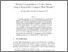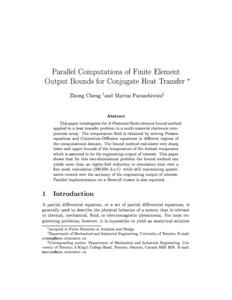Title:

# Parallel computations of finite element output bounds for conjugate heat transfer

Cheng, Zhong and Paraschivoiu, Marius (2003) Parallel computations of finite element output bounds for conjugate heat transfer. Finite Elements in Analysis and Design, 39 (7). pp. 581-597. ISSN 0168-874XPreview
Text (post-print) (application/pdf)
cp01.pdf - Accepted Version
1MB

## Abstract

This paper investigates the a posteriori finite element bound method applied to a heat transfer problem in a multi-material electronic components array. The temperature field is obtained by solving Poisson equations and convection–diffusion equations in different regions of the computational domain. The bound method calculates very sharp lower and upper bounds of the temperature of the hottest component which is assumed to be the engineering output of interest. This paper shows that for this two-dimensional problem the bound method can yield more than an 80-fold reduction in simulation time over a fine mesh calculation (330,050 d.o.f.) while still maintaining quantitative control over the accuracy of the engineering output of interest. Parallel implementation on a Beowulf cluster is also reported.

Divisions: Concordia University > Gina Cody School of Engineering and Computer Science > Mechanical and Industrial Engineering Article Yes Cheng, Zhong and Paraschivoiu, Marius Finite Elements in Analysis and Design April 2003 10.1016/S0168-874X(02)00129-4 6753 ANDREA MURRAY 02 Jul 2010 17:03 18 Jan 2018 17:29

## References:

1. M. Paraschivoiu and A.T. Patera, A hierarchical duality approach to bounds for the outputs of partial differential equations. Comput. Methods Appl. Mech. Eng. 158 (1998), pp. 389–407.

2. M. Paraschivoiu, A posteriori finite element bounds for linear-functional outputs of coersive partial differential equations and of the Stokes problem, Ph.D. Thesis, Massachusetts Institute of Technology, 1997.

3. M. Paraschivoiu, J. Peraire and A.T. Patera, A posteriori finite element bounds for linear-functional outputs of elliptic partial differential equations. Comput. Methods Appl. Mech. Eng. 150 (1997), pp. 289–321.

4. J. Peraire and A.T. Patera, Asymptotic a posteriori finite element bounds for the outputs of noncoercive problems: the Helmholtz and Burgers equations. Comput. Methods Appl. Mech. Eng. 171 (1999), pp. 77–86.

5. L. Machiels, J. Peraire and A.T. Patera, A posteriori finite element output bounds for incompressible Navier–Stokes equations; application to a natural convection problem. J. Comput. Phys. 172 (2001), pp. 401–425.

6. C. Farhat and F.-X. Roux, An unconventional domain decomposition method for efficient parallel solution of large-scale finite element systems. SIAM J. Sci. Statist. Comput. 13 1 (1992), pp. 379–396.

7. M. Paraschivoiu, A posteriori finite element output bounds in three space dimensions using the FETI method. Comput. Methods Appl. Mech. Eng. 190 (2001), pp. 6629–6640.

8. Y. Maday and A.T. Patera, Numerical analysis of a posteriori finite element bounds for linear-functional outputs. Math. Models Methods Appl. Sci. 10 5 (2000), pp. 785–799.

9. L. Machiels, M. Maday and A.T. Patera, Output bounds for reduced-order approximations of elliptic partial differential equations. Comput. Methods Appl. Mech. Eng. 190 (2001), pp. 3413–3426.

10. C. Farhat, A Lagrange multiplier based on divide and conquer finite element algorithm. J. Comput. Syst. Eng. 2 (1991), pp. 149–156.

11. C. Farhat, P.-S. Chen and J. Mandel, A scalable Lagrange multiplier based domain decomposition method for implicit time-dependent problems. Internat. J. Numer. Methods Eng. 38 (1995), pp. 3831–3854.

12. C. Farhat and F.-X. Roux, A method of finite tearing and interconnecting and its parallel solution algorithm. Comput. Methods Appl. Mech. Eng. 32 (1991), pp. 1205–1227.

13. C. Farhat and F.-X. Roux, Implicit parallel processing in structural mechanics. Comput. Mech. Adv. 2 1 (1994), pp. 1–124.

14. C. Farhat, P.-S. Chen, F. Risler and F.-X. Roux, A unified framework for accelerating the convergence of iterative substructuring methods with Lagrange multipliers. Internat. J. Numer. Methods Eng. 42 (1998), pp. 257–288.

15. D. Rixen, Substructuring and dual methods in structural analysis, Ph.D. Thesis, University of Liège, Belgium, 1997.

16. C. Farhat, K. Pierson and M. Lesoinne, The second generation FETI methods and their application to parallel solution of the large-scale linear and geometrically non-linear structural analysis problems. Comput. Methods Appl. Mech. Eng. 184 (2000), pp. 333–374.

17. R.S. Tuminaro, M. Heroux, S.A. Hutchinson, J.N. Shadid, Official aztec user's guide: version 2.1, Technical Report, Sandia National Laboratories, 1999.
All items in Spectrum are protected by copyright, with all rights reserved. The use of items is governed by Spectrum's terms of access.

Repository Staff Only: item control pageResearch related to the current document (at the CORE website)
Back to top